# Arithmetic-geometric mean process

(diff) ← Older revision | Latest revision (diff) | Newer revision → (diff)

arithmetic-geometric mean method, AGM process, AGM method, Lagrange arithmetic-geometric mean algorithm

Given two real numbers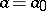and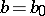, one can form the successive arithmetic and geometric means as follows: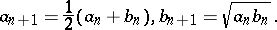(Cf. also Arithmetic mean; Geometric mean.) The sequences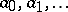and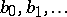rapidly converge to a common value, denoted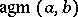and called the arithmetic-geometric mean, or sometimes the arithmetic-geometric average, of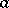and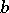. Indeed,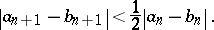This so-called AGM process is useful for computing the Jacobi elliptic functions,,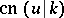,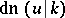, the Jacobi theta-functions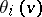(cf. also Theta-function), and the Jacobi zeta-function (see [a1], pp. 571–598, and [a4], Chap. 6; p. 663).

The number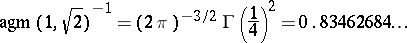is known as the Gauss lemniscate constant, or Gauss constant, [a3]. Here,denotes the Gamma-function. The lemniscate constant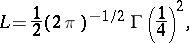which is half the total arc length of the lemniscate(cf. also Lemniscates), is closely related to the Gauss constant.

Taking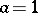,,and setting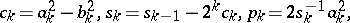one obtains a sequence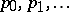that converges quadratically to, [a2] (see Pi (number)). This means that each iteration roughly doubles the number of correct digits. This algorithm is variously known as the Brent–Salamin algorithm, the Gauss–Salamin algorithm, or Salamin–Brent algorithm. There are also corresponding cubic, quartic, etc. algorithms, [a2].

How to Cite This Entry:
Arithmetic-geometric mean process. Encyclopedia of Mathematics. URL: http://encyclopediaofmath.org/index.php?title=Arithmetic-geometric_mean_process&oldid=18439
This article was adapted from an original article by M. Hazewinkel (originator), which appeared in Encyclopedia of Mathematics - ISBN 1402006098. See original article